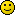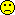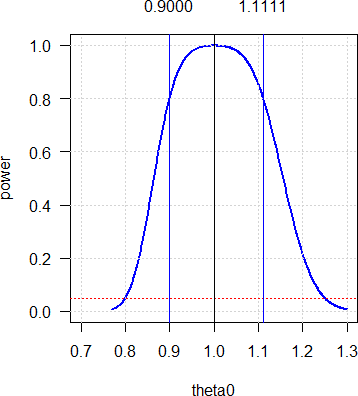BE-proff
★★

Russia,
2017-07-20 14:09

Posting: # 17577
Views: 6,564

## How to create table of results [Power / Sample Size]

Hi All,

I need to calculate sample sizes providing CV=0.26 and ratio varying from 0.85 to 1.20 with step 0,05.
How to make a script in R which returns a table with results?Thank you

Edit: Category changed. [Helmut]
ElMaestro
★★★

Belgium?,
2017-07-20 14:32

@ BE-proff
Posting: # 17578
Views: 6,027

## How to create table of results

Hi BE-proff,

try this:

library(PowerTOST) arrGMR=c(0.85, 0.9, 0.95, 1.0, 1.05, 1.10, 1.15, 1.20) myCV=0.26 N=arrGMR for (i in 1:length(arrGMR))    N[i]=sampleN.TOST(theta0=arrGMR[i], CV=myCV, targetpower=0.8) [1,7]  A=data.frame(arrGMR, N) print(A) 

you can easily put it inside a little function if the purpose is specifcally to "return" the data frame (as in, a table).

You can make the code totally fancier and totally unreadable by using sapply  or some similar function - I am sure this is what the code police recommends.I could be wrong, but...
Best regards,
ElMaestro
BE-proff
★★

Russia,
2017-07-20 15:02

@ ElMaestro
Posting: # 17579
Views: 5,993

## How to create table of results

Hi ElMaestro,

Many thanks for the code but I am just user of scriptsSo that recommendations like "insert" or "use function" are not for meBut you are right - the purpose is to make a table with resultsHelmut
★★★Vienna, Austria,
2017-07-20 15:18

@ BE-proff
Posting: # 17580
Views: 6,059

## How to create table of sample sizes / powers

Hi BE-proff,

try this (for 80 and 90% target power):

CV     <- 0.26 theta0 <- seq(0.85, 1.20, 0.05) thetas <- length(theta0) target <- c(0.8, 0.9) result <- data.frame(CV=rep(CV, thetas), GMR=sprintf("%.4f", theta0),                      n1=rep(NA, thetas), pwr1=rep(NA, thetas),                      n2=rep(NA, thetas), pwr2=rep(NA, thetas)) names(result)[3:6] <- rep(c("n", "power"), 2) for (j in seq_along(theta0)) {   x1 <- sampleN.TOST(CV=CV, theta0=theta0[j],                      targetpower=target, print=F)   x2 <- sampleN.TOST(CV=CV, theta0=theta0[j],                      targetpower=target, print=F)   result[j, 3] <- x1[["Sample size"]]   result[j, 4] <- sprintf("%.2f%%", 100*x1[["Achieved power"]])   result[j, 5] <- x2[["Sample size"]]   result[j, 6] <- sprintf("%.2f%%", 100*x2[["Achieved power"]]) } print(result, row.names=FALSE)

Should give:

   CV    GMR   n  power   n  power  0.26 0.8500 222 80.09% 308 90.15%  0.26 0.9000  60 80.18%  84 90.57%  0.26 0.9500  30 80.63%  40 90.31%  0.26 1.0000  24 80.03%  30 90.12%  0.26 1.0500  30 81.51%  40 91.01%  0.26 1.1000  52 80.76%  70 90.02%  0.26 1.1500 118 80.09% 164 90.23%  0.26 1.2000 488 80.09% 674 90.01%

Note that power curves are not symmetrical around 100%. If you assume a ∆ of 10% (without knowing whether T will be lower or higher than R), work with 0.90. The sample size will cover 1.10 as well.
Try this:

theta0 <- seq(0.85, 1, 0.05) theta0 <- unique(c(theta0, rev(1/theta0))) thetas <- length(theta0)

Should give:

   CV    GMR   n  power   n  power  0.26 0.8500 222 80.09% 308 90.15%  0.26 0.9000  60 80.18%  84 90.57%  0.26 0.9500  30 80.63%  40 90.31%  0.26 1.0000  24 80.03%  30 90.12%  0.26 1.0526  30 80.63%  40 90.31%  0.26 1.1111  60 80.18%  84 90.57%  0.26 1.1765 222 80.09% 308 90.15%

Do you get the idea?

Cheers,
Helmut SchützThe quality of responses received is directly proportional to the quality of the question asked. ☼
Science Quotes
BE-proff
★★

Russia,
2017-07-20 16:00

@ Helmut
Posting: # 17582
Views: 5,947

## How to create table of sample sizes / powers

Hi Helmut,

No, I didn't catch an idea.Do you think that absence of symmetry critical?
Helmut
★★★Vienna, Austria,
2017-07-20 17:12

@ BE-proff
Posting: # 17583
Views: 6,064

## Symmetrical in log-scale

Hi BE-proff,

» No, I didn't catch an idea.» Do you think that absence of symmetry critical?

Power curves are asymmetrical around 1 in linear scale (as is the acceptance range) but symmetrical around 0 in logarithmic scale (AR: ±0.2231). Try this (CV 0.26, GMR 0.9, n 60 for target power 80%):

library(PowerTOST) CV     <- 0.26 GMR    <- 0.90 theta0 <- seq(1/1.3, 1.3, length.out=501) n      <- sampleN.TOST(CV=0.26, theta0=GMR, print=FALSE)[["Sample size"]] power  <- numeric(length(theta0)) for (j in seq_along(theta0)) {   power[j] <- power.TOST(CV=CV, theta0=theta0[j], n=n) } dev.new(record=TRUE) op     <- par(no.readonly=TRUE) par(pty="s", ask=TRUE) # linear scale # plot(theta0, power, type="l", las=1, col="blue", lwd=2,      xlim=c(2-max(theta0), max(theta0)), ylim=c(0, 1)) grid() abline(h=0.05, col="red", lty="dotted") abline(v=c(1, GMR, 1/GMR), col=c("black", rep("blue", 2))) mtext(sprintf("%.4f", c(GMR, 1/GMR)), side=3,       at=c(GMR, 1/GMR), line=1) # logarithmic scale # plot(log(theta0), power, type="l", las=1, col="blue", lwd=2,      xlim=c(-1, +1)*max(abs(range(log(theta0)))), ylim=c(0, 1)) abline(h=0.05, col="red", lty="dotted") grid() abline(h=0.05, col="red", lty="dotted") abline(v=log(c(1, GMR, 1/GMR)), col=c("black", rep("blue", 2))) mtext(sprintf("%+.4f", log(c(GMR, 1/GMR))), side=3,       at=log(c(GMR, 1/GMR)), line=1) par(op)

Press or click in the graphics window to go to the next plot. To switch between plots navigate to the graphics window and use / to switch plots.The power curve is positively skewed. For any –∆ you get the same power at 1/∆. In the example: For GMR 1.1111 as for GMR 0.90 since 1/0.90 = 1.1111. In many protocols I read “planned for a T/R-ratio of 0.90 to 1.10”. Nope. If the sample size estimation was done for 0.90 it covers anything up to 1.1111. Or the other way ’round: If it was done for 1.10 it would cover anything down to only 1/1.1 = 0.9091 (and not 0.90). Hence, the latter is stupid – unless you know (!) that the GMR will be >1. If you don’t know the sign of ∆, always plan for a GMR <1 and you will be on the safe side.

The second part of the script demonstrates that in log-scale (where the entire analysis is done) everything is symmetrical indeed:Hope that helps.

Cheers,
Helmut SchützThe quality of responses received is directly proportional to the quality of the question asked. ☼
Science Quotes
BE-proff
★★

Russia,
2017-07-24 11:42

@ Helmut
Posting: # 17599
Views: 5,838

## Symmetrical in log-scale

Hi Helmut,

Many thanks for clarification!Could you please tell how to add study design tolibrary(PowerTOST) CV     <- 0.26 theta0 <- seq(0.85, 1.20, 0.05) thetas <- length(theta0) target <- c(0.8, 0.9) result <- data.frame(CV=rep(CV, thetas), GMR=sprintf("%.4f", theta0),                      n1=rep(NA, thetas), pwr1=rep(NA, thetas),                      n2=rep(NA, thetas), pwr2=rep(NA, thetas)) names(result)[3:6] <- rep(c("n", "power"), 2) for (j in seq_along(theta0)) {   x1 <- sampleN.TOST(CV=CV, theta0=theta0[j],                      targetpower=target, print=F)   x2 <- sampleN.TOST(CV=CV, theta0=theta0[j],                      targetpower=target, print=F)   result[j, 3] <- x1[["Sample size"]]   result[j, 4] <- sprintf("%.2f%%", 100*x1[["Achieved power"]])   result[j, 5] <- x2[["Sample size"]]   result[j, 6] <- sprintf("%.2f%%", 100*x2[["Achieved power"]]) } print(result, row.names=FALSE)
Helmut
★★★Vienna, Austria,
2017-07-24 18:18

@ BE-proff
Posting: # 17600
Views: 5,784

## RTFM

Hi BE-proff,

no need to copypaste my entire code.

» Could you please tell how to add study design toPlease do your homework and RTFM:

library(PowerTOST) help(sampleN.TOST) help(known.designs)

Online:

sampleN.TOST known.designs 

Add the design-string (as provided in second column of known.designs()) as an argument to sampleN.TOST(...) in the two lines starting with x1 <- and x2 <-
Easy.

Cheers,
Helmut SchützThe quality of responses received is directly proportional to the quality of the question asked. ☼
Science Quotes
Bioequivalence and Bioavailability Forum |  Admin contact
19,694 posts in 4,181 threads, 1,355 registered users;
online 12 (0 registered, 12 guests [including 7 identified bots]).
Forum time (Europe/Vienna): 13:33 CEST

A central lesson of science is that to understand complex issues
(or even simple ones), we must try to free our minds of dogma and
to guarantee the freedom to publish, to contradict, and to experiment.
Arguments from authority are unacceptable.    Carl Sagan

The BIOEQUIVALENCE / BIOAVAILABILITY FORUM is hosted byIng. Helmut Schütz